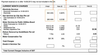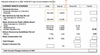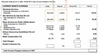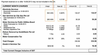# Utility bills Flashcards Preview

## Maths in Practical Situation II > Utility bills > Flashcards

Flashcards in Utility bills Deck (11):
1

2

3

4

5

6

## Calculate the cost the electricity used### 504 * 0.2670 = \$134.577

## Calculate the cost the gas used### 260 * 0.2126 = \$55.288

## Calculate the water conservation tax### \$21.76 * 30/100 = \$6.539

## Calculate the total charges without GST### \$134.57 + \$55.28 + \$32.58 + \$4.03 + \$6.53 = \$232.9910

## Calculate the GST### \$232.99 * 7/100 = \$16.3011

## Calculate the Final bill### \$232.99 + \$16.30 = \$249.29